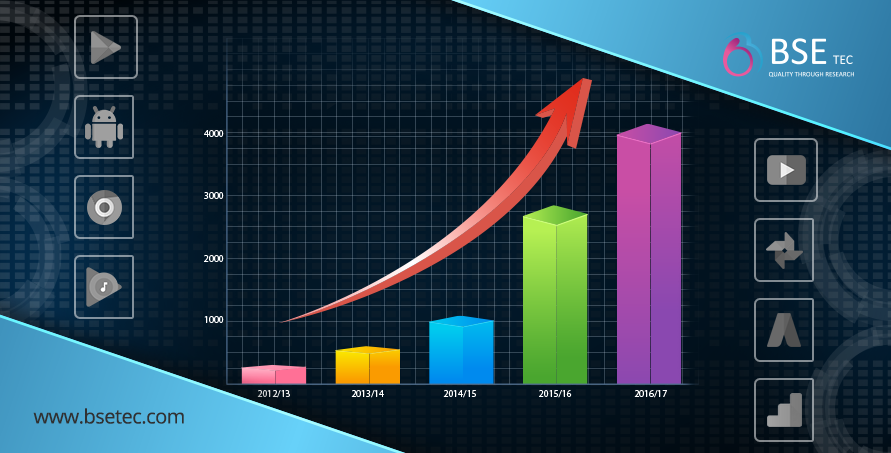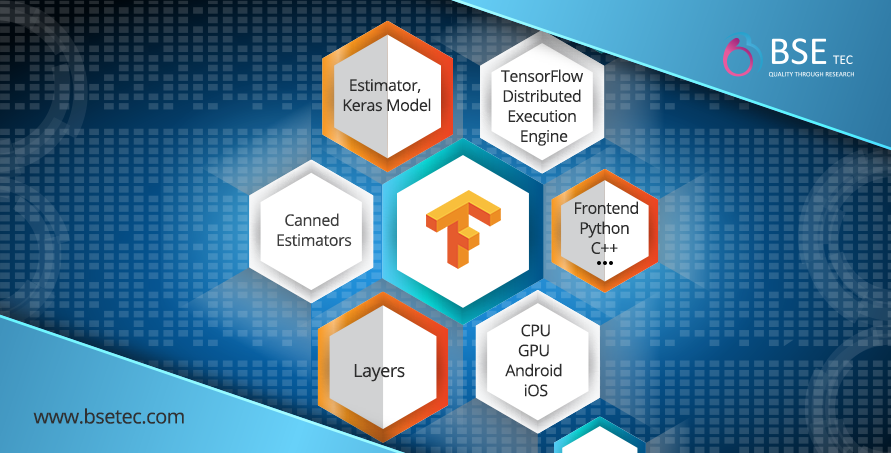# Introduction to TensorFlow

TensorFlow is useful to have a mental model for how the system behaves and server behaves, but mostly you can forget everything and use the high-level wrappers. If your job is just to use machine learning. Machine learning algorithms out of the shelf. If you are a researcher or developer of machine learning algorithms, you should also know low-level TensorFlow.

#### What is TensorFlow?

TensorFlow is open source Machine Learning library, it was originally developed by Google and it has been open source since November 2015. TensorFlow is especially used for deep learning. Non-computer science people can also run some of the mathematical simulations or any complex mathematical calculations using TensorFlow. Core TensorFlow is not really tied to machine learning, using TensorFlow any mathematical computations can be defined. TensorFlow can be used for research as well as in production environment. Startup companies may thinking of using machine learning can think of using TensorFlow. TensorFlow codes are available in community so that people can experiment that code later.

There is some meaning in the name TensorFlow, it is combination of two words, Tensor and Flow. In this context Tensor is a multidimensional array and Flow is nothing but graph of operations. Every mathematical computations is expressed as a graph of operations in TensorFlow. So that the nodes in the graph are operations, and edges are nothing but tensors. So tensors flow into operations, imagine like a vector matrix multiplications. Vector and Matrix will be the input and Multiple will be the operations.Google is using TensorFlow internally very heavily. Google uses TensorFlow in many of its products.#### Tools for Running in different environments:

Really Good part about TensorFlow is this, TensorFlow can execute operations on various hardware platforms like CPU, GPU, Android, IOS, Raspberry Pi etc… even we can consider TensorFlow in case of writing the embedded systems.

How to achieve this,
There is something called TensorFlow Distributed Execution Engine. Generally codes are written either in Python or C++ or JAVA. TensorFlow Distributed Execution Engine takes the code written in python and converts it into the hardware instruction set. In Layers we have all the reusable machine learning components and then we have estimators, Estimators is a framework for any machine learning model and used for writing new machine learning algorithms. Then comes the Canned Estimators supports, liner regression, logistics regression and neural networks out of the box.TensorFlow provides APIs for beginners also for experts to develop desktop apps, mobile apps, web apps, and cloud. The high-level Keras API is a building blocks to create and train the deep learning models.